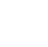# LOOPS:

A Loop executes the sequence of statements many times until the stated condition becomes false. A loop consists of two parts, a body of a loop and a control statement. The purpose of the loop is to repeat the same code several times.

## 1. while loop :

A while loop statement in Java programming language repeatedly executes a target statement as long as a given condition is true. When executing, if the boolean_expression result is true, then the actions inside the loop will be executed. When the condition becomes false, the loop will exit.

Syntax:

while(Boolean_expression) {
// Statements
}

Example:

public class Looping {

public static void main(String args[]) {
int x = 10;

while( x < 15 ) {
System.out.print("value x : " + x );
x++;
System.out.print("\\n");
}
}
}
/*EXPLANATION:
The loop executes until the value of x is less than 15, the value of x is incremented by 1. When the condition is false the loop exit */

/*OUTPUT

value x : 10
value x : 11
value x : 12
value x : 13
value x : 14 */

## 2. do while loop  :

A do-while loop is similar to a while loop with one exception that it executes the statements inside the body of the do-while before checking the condition. If the Boolean expression is true, the statements in the loop execute again. This process repeats until the Boolean expression is false.

Syntax:

do {
// Statements
}while(Boolean_expression);

Expressions:

public class Looping {

public static void main(String args[]) {
int x = 10;

do {
System.out.print("value x : " + x );
x++;
System.out.print("\\n");
}while( x < 15 );
}
}
/*EXPLANATION:
The control passes to the body of the loop and then checks the condition.
If the condition is less than 15 loop executes, and then the value of x is incremented
by 1.When the condition false the loop terminates. */

/*OUTPUT

value x : 10
value x : 11
value x : 12
value x : 13
value x : 14 */

## 3. for loop

A for loop ( finite loop ) is a repetition control structure that allows you to efficiently write a loop that needs to be executed a specific number of times. A for loop is useful when you know how many times a task is to be repeated.

Syntax:

for(initialization; Boolean_expression; update) {
// Statements
}

Example:

public class Looping {

public static void main(String args[]) {

for(int x = 10; x < 15; x = x + 1) {
System.out.print("value x : " + x );
System.out.print("\\n");
}
}
}
/*EXPLANATION:
The loop executes until the value of x is less than 15,
when the condition is false the loop exit*/

/* OUTPUT

value x : 10
value x : 11
value x : 12
value x : 13
value x : 14 */

## Jump Statements:

Jump statements are used to unconditionally transfer program control from one point to elsewhere in the program. Jump statements are primarily used to interrupt loops or switch-case instantly. Java supports three jump statements: break, continue, and return.

## i) break statement:

The break construct is used to break out of the middle of loops: for, do, or while loop. When a break statement is encountered, execution of the current loops immediately stops and resumes at the first statement following the current loop.

Example:

public class BreakStatement{
public static void main(String args[]) {
System.out.println("Welcome to ShapeAI");
for (int i = 1; i <= 10; i++) {
System.out.println("i = "+i);
if (i == 5) {
System.out.println("\\nBye");
break;//hence break statement is used to terminate the loop
}
}
}
}
/*OUTPUT:
Welcome to ShapeAI
i = 1
i = 2
i = 3
i = 4
i = 5

Bye */

## ii) continue Statement:

The continue statement also skips the remaining statements of the body of the loop where it is defined but instead of terminating the loop, the control is transferred to the beginning of the loop for the next iteration. The loop continues until the test condition of the loop becomes false.

Example:

public class ContinueStatement{
public static void main(String args[]) {
int i;
for (i = 1; i <= 10; i++) {
if (i == 5)
continue; // continue statement breaks the loop continues until the test condition of the loop becomes false
System.out.print(i + " ");
}
}
}
/*OUTPUT:
1 2 3 4 6 7 8 9 10 */

## iii) return Statement:

A return statement ends the execution of a function, and returns control to the calling function. Execution resumes in the calling function at the point immediately following the call. A return statement can return a value to the calling function.

Example:

public class ReturnStatement {
int display() {
return 5;
}
public static void main(String[] args) {
ReturnStatement e = new ReturnStatement();
System.out.println(e.display());
}
}

/*OUTPUT:
5  */